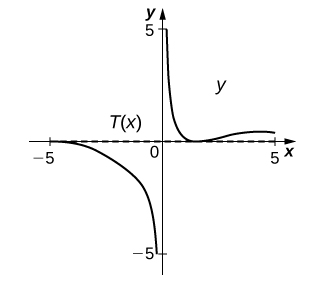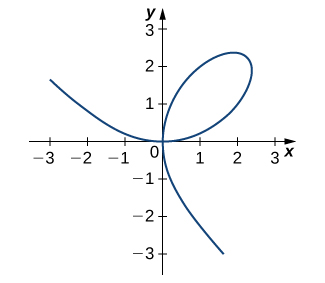$$\newcommand{\id}{\mathrm{id}}$$ $$\newcommand{\Span}{\mathrm{span}}$$ $$\newcommand{\kernel}{\mathrm{null}\,}$$ $$\newcommand{\range}{\mathrm{range}\,}$$ $$\newcommand{\RealPart}{\mathrm{Re}}$$ $$\newcommand{\ImaginaryPart}{\mathrm{Im}}$$ $$\newcommand{\Argument}{\mathrm{Arg}}$$ $$\newcommand{\norm}{\| #1 \|}$$ $$\newcommand{\inner}{\langle #1, #2 \rangle}$$ $$\newcommand{\Span}{\mathrm{span}}$$

# 3.8 E: Implicit Differentiation Exercises

$$\newcommand{\vecs}{\overset { \rightharpoonup} {\mathbf{#1}} }$$ $$\newcommand{\vecd}{\overset{-\!-\!\rightharpoonup}{\vphantom{a}\smash {#1}}}$$$$\newcommand{\id}{\mathrm{id}}$$ $$\newcommand{\Span}{\mathrm{span}}$$ $$\newcommand{\kernel}{\mathrm{null}\,}$$ $$\newcommand{\range}{\mathrm{range}\,}$$ $$\newcommand{\RealPart}{\mathrm{Re}}$$ $$\newcommand{\ImaginaryPart}{\mathrm{Im}}$$ $$\newcommand{\Argument}{\mathrm{Arg}}$$ $$\newcommand{\norm}{\| #1 \|}$$ $$\newcommand{\inner}{\langle #1, #2 \rangle}$$ $$\newcommand{\Span}{\mathrm{span}}$$ $$\newcommand{\id}{\mathrm{id}}$$ $$\newcommand{\Span}{\mathrm{span}}$$ $$\newcommand{\kernel}{\mathrm{null}\,}$$ $$\newcommand{\range}{\mathrm{range}\,}$$ $$\newcommand{\RealPart}{\mathrm{Re}}$$ $$\newcommand{\ImaginaryPart}{\mathrm{Im}}$$ $$\newcommand{\Argument}{\mathrm{Arg}}$$ $$\newcommand{\norm}{\| #1 \|}$$ $$\newcommand{\inner}{\langle #1, #2 \rangle}$$ $$\newcommand{\Span}{\mathrm{span}}$$

## 3.8: Implicit Differentiation

For the following exercises, use implicit differentiation to find $$\frac{dy}{dx}$$.

300) $$x^2−y^2=4$$

301) $$6x^2+3y^2=12$$

$$\frac{dy}{dx}=\frac{−2x}{y}$$

Solution: $$\frac{dy}{dx}=\frac{−2x}{y}$$

302) $$x^2y=y−7$$

303) $$3x^3+9xy^2=5x^3$$

$$\frac{dy}{dx}=\frac{x}{3y}−\frac{y}{2x}$$

304) $$xy−\cos(xy)=1$$

305) $$y\sqrt{x+4}=xy+8$$

$$\frac{dy}{dx}=\frac{y−\frac{y}{2\sqrt{x+4}}}{\sqrt{x+4}−x}$$

306) $$−xy−2=\frac{x}{7}$$

307) $$y\sin(xy)=y^2+2$$

$$\frac{dy}{dx}=\frac{y^2\cos(xy)}{2y−\sin(xy)−xy\cosxy}$$

308) $$(xy)^2+3x=y^2$$

309) $$x^3y+xy^3=−8$$

$$\frac{dy}{dx}=\frac{−3x^2y−y^3}{x^3+3xy^2}$$

For the following exercises, find the equation of the tangent line to the graph of the given equation at the indicated point. Just for observation, use a calculator or computer software to graph the function and the tangent line.

310) $$[T] x^4y−xy^3=−2,(−1,−1)$$

311) $$[T] x^2y^2+5xy=14,(2,1)$$

$$y=−\frac{1}{2}x+2$$312) $$[T] tan(xy)=y,(\frac{π}{4},1)$$

313) $$[T] xy^2+sin(πy)−2x^2=10,(2,−3)$$

$$y=\frac{1}{π+12}x−\frac{3π+38}{π+12}$$314) $$[T] \frac{x}{y}+5x−7=−\frac{3}{4}y,(1,2)$$

315) $$[T] xy+\sin(x)=1,(\frac{π}{2},0)$$

$$y=0$$316) [T] The graph of a folium of Descartes with equation $$2x^3+2y^3−9xy=0$$ is given in the following graph.a. Find the equation of the tangent line at the point $$(2,1)$$. Graph the tangent line along with the folium.

b. Find the equation of the normal line to the tangent line in a. at the point $$(2,1)$$.

317) For the equation $$x^2+2xy−3y^2=0,$$

a. Find the equation of the normal to the tangent line at the point $$(1,1)$$.

b. At what other point does the normal line in a. intersect the graph of the equation?

$$a. y=−x+2 b. (3,−1)$$

318) Find all points on the graph of $$y^3−27y=x^2−90$$ at which the tangent line is vertical.

319) For the equation $$x^2+xy+y^2=7$$,

a. Find the $$x$$-intercept(s).

b.Find the slope of the tangent line(s) at the x-intercept(s).

c. What does the value(s) in b. indicate about the tangent line(s)?

$$a. (±7√,0) b. −2$$ c. They are parallel since the slope is the same at both intercepts.

320) Find the equation of the tangent line to the graph of the equation $$sin^{−1x}+sin^{−1}y=\frac{π}{6}$$ at the point $$(0,\frac{1}{2})$$.

321) Find the equation of the tangent line to the graph of the equation $$tan^{−1}(x+y)=x^2+\frac{π}{4}$$ at the point $$(0,1)$$.

$$y=−x+1$$

322) Find $$y′$$ and $$y''$$ for $$x^2+6xy−2y^2=3$$.

323) [T] The number of cell phones produced when $$x$$ dollars is spent on labor and $$y$$ dollars is spent on capital invested by a manufacturer can be modeled by the equation $$60x^{3/4}y^{1/4}=3240$$.

a. Find $$\frac{dy}{dx}$$ and evaluate at the point $$(81,16)$$.

b. Interpret the result of a.

$$a. −0.5926$$ b. When $81 is spent on labor and$16 is spent on capital, the amount spent on capital is decreasing by $0.5926 per$1 spent on labor.

324) [T] The number of cars produced when x dollars is spent on labor and y dollars is spent on capital invested by a manufacturer can be modeled by the equation $$30x^{1/3}y^{2/3}=360$$.

(Both $$x$$and $$y$$ are measured in thousands of dollars.)

a. Find $$\frac{dy}{dx}$$ and evaluate at the point $$(27,8)$$.

b. Interpret the result of a.

325) The volume of a right circular cone of radius $$x$$ and height $$y$$ is given by $$V=\frac{1}{3}πx^2y$$. Suppose that the volume of the cone is $$85πcm^3$$. Find $$\frac{dy}{dx}$$ when $$x=4$$ and $$y=16$$.

$$−8$$
326) For the following exercises, consider a closed rectangular box with a square base with side $$x$$ and height $$y$$.
Find an equation for the surface area of the rectangular box, $$S(x,y)$$.
327) If the surface area of the rectangular box is 78 square feet, find $$\frac{dy}{dx}$$ when $$x=3$$ feet and $$y=5$$ feet.
$$−2.67$$